5. GALACTIC STRUCTURE

The preceding sections describe the path by which gas achieves sufficiently high density to allow a galaxy to form. The next significant question is what structure that galaxy will have. In the following section we will review the process of disk and spheroid formation and make quantitative estimates of the sizes of these structures.

Disks are a common astrophysical phenomenon and galactic disks owe their origins to the same fundamental process as other astrophysical disks: conservation of angular momentum in a system collapsing under gravity eventually leading to arrest of the collapse by rotational support. Understanding the physical properties of galactic disks therefore requires knowledge of their angular momentum content and the gravitational potential in which they form.

The angular momentum of the gas which will eventually form a galactic disk arises in the same way as that of dark matter halos, namely from tidal torques from surrounding large scale structure (Hoyle 1949) 12. The magnitude of the angular momentum content of the baryonic component of a halo is close to being a scaled version of that of the dark matter - for example, van den Bosch et al. (2002) find that the distributions of spin parameters and angular momentum distributions within individual halos for dark matter and non-radiative gas are very similar. They find that there is, however, a significant (typically 30°) misalignment between the dark matter and gas angular momenta vectors 13. Numerical hydrodynamical studies show that the angular momenta vectors of galactic disks are well aligned with the angular momenta of the inner regions of dark matter halos (Bett et al. 2009, Hahn et al. 2010).

The distribution of the angular momentum is less well studied but recent investigations (Sharma and Steinmetz 2005) using non-radiative hydrodynamical simulations have shown that the differential distribution of specific angular momenta, j, is given by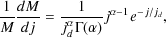(53)

whereis the gamma function, M is the total mass of gas, jd = jtot /and jtot is the mean specific angular momentum of the gas. Sharma and Steinmetz (2005) find that the simulated halos have a median value of= 0.89. The fraction of mass with specific angular momentum less than j is then given by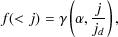(54)

whereis the incomplete gamma function.

We have assumed so far that the angular momentum of the pre-galactic gas is conserved during collapse. This may not be precisely true and indeed did not seem to be in earlier generations of hydrodynamical simulations which typically found that disk galaxies lost significant fractions of their angular momentum and, as a result, were too small (Navarro and Benz 1991; Navarro and White 1994; Navarro et al. 1995). However, more recent simulations (Thacker and Couchman 2001; Steinmetz and Navarro 2002) do show approximate conservation of angular momentum (most likely due to the inclusion of effective feedback in these later generations of simulations) and, furthermore, conservation of angular momentum leads to disks with sizes comparable to those observed - certainly the gas cannot lose a significant fraction of its angular momentum if it is to form a disk of comparable size to observed galactic disks. Confirmation of these ideas has recently been made by Zavala et al. (2008) who convincingly show in N-body+hydrodynamical simulations that the particular feedback prescription used can lead to the formation of a disk dominated or spheroid dominated galaxy in the same dark matter halo, with stronger feedback leading to a disk galaxy. The angular momentum of the mass in the disk galaxy tracks that of the dark matter halo as a whole, growing as expected during the linear regime and remaining nearly constant after halo collapse. With weaker feedback a spheroid forms instead. Its angular momentum also grows during the linear regime but then declines rapidly (as does that of the inner regions of the dark matter halo) due to angular momentum transfer from dense, progenitor blobs to the surrounding, diffuse dark matter. Feedback processes are clearly of crucial importance here - for example, Wise and Abel (2008b) show that feedback during the formation of protogalaxies at early times can effectively boost the angular momentum content of such systems by factors of three to five. This is achieved via forces associated with HII region and SNe blast waves which produce torques much stronger than the usual cosmological torques.

The physics which determines the sizes of galaxy disks was originally described by Fall and Efstathiou (1980) and later expanded upon by many authors (see, in particular, Mo et al. 1998, Mao et al. 1998).

Once the angular momentum distribution of that part of the baryonic component of the halo which cools to form the galaxy is known, finding the structure of the resulting rotationally supported disk is reduced to solving the following equation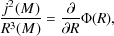(55)

where j(M) is the specific angular momentum enclosing mass M and this equation is solved for R(M). The potential is a sum of the self-gravity of the disk and that of any external potential (dark matter halo and bulge for example) which may have responded adiabatically to the formation of the disk. For a thin disk, the potential is generically given by (Binney and Tremaine 2008)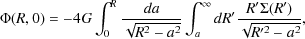(56)

which, for an exponential surface density profile 14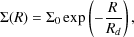(57)

simplifies to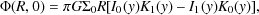(58)

where y = R / 2Rd and I0, I1, K0 and K1 are Bessel functions. Knowing the functional form of j(M) it is possible to solve for R(M) and therefore the density profile of the disk. Often, for simplicity, a particular form for the density (e.g. exponential) is assumed which leaves a single free parameter (the scale length) to be solved for.

A significant complication to this picture arises from the fact that the external potential in which the disk forms is likely to change in response to the formation of the disk. If the disk forms slowly, such that the timescale for changes in the potential greatly exceeds the dynamical time of the dark matter halo, then we can use adiabatically invariant quantities (Binney and Tremaine 2008) to estimate the response of the halo to the forming disk. The original formulation of this argument for galaxies was given by Blumenthal et al. (1986) and has been used extensively ever since. In this simplified picture, the dark matter particles are considered to be on circular orbits in a spherical potential. In this case, the only adiabatic invariant whose corresponding angle variable has a non-infinite period is the magnitude of the angular momentum, L. Prior to the formation of any galaxy, this angular momentum is given by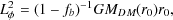(59)

where MDM(r) is the mass of dark matter within radius r, r0 is the initial radius of the circular orbit and we have assumed that baryons are present in the halo at the universal fraction, fb(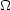b / [b +CDM]) and distributed as the dark matter. If Lis conserved during the formation of a galaxy, then, at any point after galaxy formation has begun we have(60)

where M'(r) is the mass of dark matter enclosed within radius r after responding to the forming galaxy, Mgal(r) is the mass of the galaxy within that same radius 15, f'b is the baryon fraction remaining uncondensed into the galactic phase and rf is the radius of the new circular orbit for this dark matter particle. If we assume no shell crossing, then M'DM(rf) = MDM(r0). Given a knowledge of the mass and density distribution of the galaxy this allows us to solve for rf as a function of the initial radius r0.

This approach makes several simplifying assumptions, including adiabatic growth, a spherical halo and circular orbits for dark matter particles. Many of these assumptions have yet to be adequately tested. However, recent work has attempted to address the last of these. With a more realistic distribution function for the dark matter, the particles will occupy a range of orbital eccentricities. In a spherical potential, the angular momentum is joined by a second adiabatic invariant, the radial action: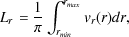(61)

where the integral is taken along the orbit of a particle from its pericenter to apocenter. The effects of taking into account this second invariant and a physically reasonable orbital distribution have been explored by Gnedin et al (2004).. Gnedin et al (2004). find that the simple model described above systematically overpredicts the degree of contraction of the halo. However, they find that a simple modification in which the combination M(r)r in the above is replaced with M()r, whereis the orbit-averaged radius, approximately accounts for the orbital eccentricities and gives good agreement with numerical simulations. Gnedin et al (2004). further find that the orbit-averaged radius in cosmological halos can be well fit by the relation/ rvir = A (r / rvir)w with A = 0.85 ± 0.05 and w = 0.8 ± 0.02. More recent simulations (Gustafsson et al. 2006) support this picture, but show that the optimal values of A and w vary from galaxy to galaxy and seem to depend on the formation history (i.e. on the adopted rules for star formation and feedback; see also Abadi et al. 2009). This suggests that the process of halo response to galaxy growth is more complicated than is captured by these simple models, perhaps because the assumed invariants are not precisely invariant in non-spherical potentials or perhaps because galaxy growth is not sufficiently slow to be truly adiabatic.

Galaxy disks are, of course, not razor thin. The origins of the vertical extent of galaxy disks remain a topic of active research but various possibilities are currently considered seriously:

1. External origins (accretion):

1. The hierarchical nature of galaxy formation implies that a galactic disk can expect to accrete pre-existing, smaller stellar systems frequently during its life. The stars from these smaller galaxies are often found in numerical simulations to form a thickened disk structure, in the same plane as the pre-existing disk of the galaxy (Abadi et al. 2003; Villalobos and Helmi 2008). This is to be expected as dynamical friction will tend to drag orbiting satellites into the plane of the disk where tidal forces will proceed to shred the satellite, leaving its stars orbiting in the plane of the disk.
2. Early, chaotic gas accretion: In a slightly different scenario, Brook et al. (2004) find, in numerical simulations of a forming galaxy, that many of the thick disk stars form from accreted gaseous systems during an early, chaotic period of merging.

2. Internal origins (dynamical heating):

1. Dark matter substructure: Building upon earlier works (Toth and Ostriker 1992; Quinn et al. 1993; Sellwood et al. 1998; Velazquez and White 1999), in recent years there have been numerous studies focused on the question of whether relatively thin galactic disks can survive in the rather violent environment of a hierarchically formed dark matter halo (Font et al. 2001; Benson et al. 2004; Kazantzidis et al. 2008; Read et al. 2008; Kazantzidis et al. 2009; Purcell et al. 2009). A careful treatment of dark matter substructure orbital evolution and the build-up of the substructure population over time is required to address this problem. The consensus conclusion from these studies is that galactic disks can survive in the currently accepted cold dark matter cosmogony, but that interactions with orbiting dark matter substructure must be a significant contribution to the thickening of galaxy disks. The heating is dominated by the most massive dark matter substructures and so is a rather stochastic process, depending on the details of the merger history of each galaxy's dark matter halo and the orbital properties of those massive substructures.

2. Molecular clouds: Massive molecular clouds can gravitationally scatter stars that happen to pass by them, effectively transforming some of their orbital energy in the plane of the galaxy disk into motion perpendicular to that plane, thereby effectively thickening the disk (Spitzer and Schwarzschild 1953; Lacey 1984).

3. Spiral arms: Spiral density waves can also act as scatterers of stars, but predominantly increase the stellar velocity dispersion in the plane of the galaxy, resulting in little thickening (Jenkins and Binney 1990; Minchev and Quillen 2006).

Most likely, a combination of these processes is at work. A recent review of these mechanisms is given by Wen and Zhao (2004).

Spiral arms and other non-axisymmetric features such as bars in galactic disks are a visually impressive reminder that these systems possess interesting dynamics. The basic theory of spiral arms, that they are density waves, was first proposed by Lin and Shu (1964; see also Marochnik and Suchkov 1996, Binney and Tremaine 2008) and has come to be widely accepted. From our current standpoint, the question in which we are interested in is whether these perturbations to an otherwise smooth disk are stable or unstable and, if unstable, how they affect the evolution of the galaxy as a whole.

Toomre (1964; see also Goldreich and Lynden-Bell 1965) derived an expression for the local stability of thin disks to axisymmetric modes in the tight-winding approximation which turns out to be extremely useful (and often approximately correct even in regimes where its assumptions fail). Disks will be unstable to axisymmetric modes if Q < 1 where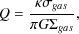(62)

where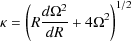(63)

is the epicyclic frequency andis the angular frequency of the disk, for a gaseous disk of surface densitygas and velocity dispersiongas, and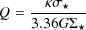(64)

for a stellar disk of surface density* and velocity dispersion*. For a disk consisting of two components, gas and stars, a joint stability analysis was carried out by Jog and Solomon (1984). Efstathiou (2000; see also Bertin and Romeo 1988) solves the resulting cubic equation for the most unstable mode and finds a criterion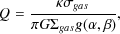(65)

whereand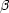are the ratio of stellar to gas velocity dispersions and surface densities respectively and the function g(,) is as given by Efstathiou (2000) and is shown in Fig. 7. Wang and Silk (1994) also show that the stability of a two-component disk can be approximated by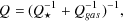(66)

while a more general criterion taking into account the thickness of the disk was found by Romeo (1992) and Romeo (1994).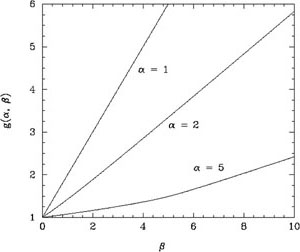Figure 7. The function g(,) appearing in eqn. (65). g(,) is shown as a function of(the ratio of stellar to gas velocity dispersions) for various values of(the ratio of stellar to gas surface density). Reproduced, with permission, from Efstathiou (2000).

Toomre's criterion applies to local perturbations. Perturbations on the scale of the disk can occur also. Study of these global instabilities is less amenable to analytic treatment (since one can no longer ignore contributions from distant parts of the disk). Significant work on this subject began in the 1970's. The classic result from that time is due to Ostriker and Peebles (1973) who found that rapidly rotating, self-gravitating stellar systems would become violently unstable to non-axisymmetric m = 2 modes if T / |W|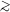0.14 (for a stellar system) or 0.27 (for a fluid system) where T and W are the kinetic and potential energies of the system. Such systems can be unstable to global perturbations even if they satisfy Toomre's stability criterion. These values were approximately confirmed by numerous numerical studies, but also challenged by others (see Christodoulou et al. 1995b for a discussion of this). Later work (Christodoulou et al. 1995a) has demonstrated that T / |W| is not a sufficiently general parameter to determine stability in all systems. More recent studies have proposed improved stability criteria. For example Christodoulou et al. (1995b) find that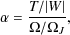(67)

whereJ is the Jeans frequency in the radial direction andis the mean angular velocity of the system, is a much better indicator of stability. This can be approximated by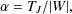(68)

where TJ = LJ / 2 and L is the total angular momentum of the system. Christodoulou et al. (1995b) found that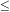0.254-0.258 is required for stability in stellar systems while0.341-0.354 is required for gaseous systems. Christodoulou et al. (1995b) also demonstrate that this criterion is approximately equivalent to the alternative form found by Efstathiou et al. (1982) on the basis of numerical simulations of exponential stellar disks: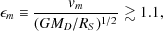(69)

and show that an equivalent result for gaseous disks would replace the 1.1 with 0.9. A much more extensive review of disk stability is given by Sellwood (2010).

Global instabilities most likely lead to the formation of a very strong bar which effectively disrupts the disk leaving, after a few dynamical times, a boxy/peanut bulge or a disky bulge (Athanassoula 2008). This may therefore be a possible formation scenario for pseudo-bulges (Kormendy and Kennicutt 2004), i.e. bulges formed through secular processes in the disk (see Section5.2.2) rather than as the result of a merger event (see Section 5.2.1).

The presence of perturbations in the disk can also affect its structure in less dramatic but still significant ways. (Once again, the work by Sellwood 2010 gives a more in depth review of these subjects and is highly recommended.) For example, numerical simulations by Roskar et al. (2008b; see also Roskar et al. 2008a) show that a significant fraction of stars in galaxy disks undergo large migrations in radius (as shown in Fig. 9) due to resonant scattering from spiral arms. The resonant scattering of a star initially on an approximately circular orbit tends to change its energy and angular momentum in such a way as to keep it in a circular orbit, but to change the radius of that orbit. In a frame corotating with the spiral perturbation there is an energy-like invariant quantity know as Jacobi's integral, EJ, defined as (Binney and Tremaine 2008)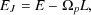(70)

where E and L are the energy and angular momentum of an orbit respectively andp is the pattern speed of the perturbation. This implies that changes in energy and angular momentum are related by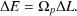(71)

As shown in Fig. 8 the slope of the E - L relation for circular orbits at the corotation resonance isp. As such, stars initially on circular orbits near the corotation resonance will tend to be scattered into approximately circular orbits. Roskar et al. (2008b) show that this phenomenon can lead to the formation of an outer stellar disk with a steeper exponential decline than the inner disk and formed entirely of stars scattered from the inner regions of the galaxy. This process also smooths out age and metallicity gradients in disks that otherwise form inside out and so tend to be older and less metal rich in the center.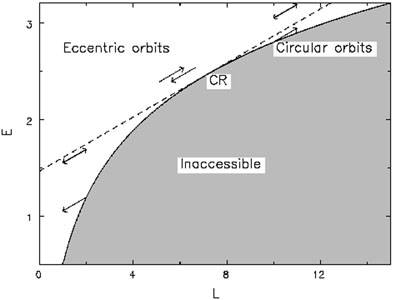Figure 8. The Lindblad diagram showing allowed regions of the energy-angular momentum plane (white, with forbidden regions shaded gray) bounded by the curve corresponding to circular orbits. Arrows indicate how scattering from spiral density waves moves stars in this diagram. At the corotation resonance (CR) this motion parallels the circular orbit curve, implying that scattering at corotation of stars initially on circular orbits leaves those stars on approximately circular orbits. Reproduced, with permission, from Sellwood and Binney (2002).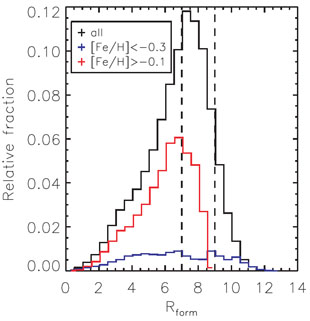Figure 9. The distribution of formation radii, Rform (in kpc), for stars in the Solar neighborhood. The black histogram shows the distribution from all stars while the red and blue histograms show the contributions from high and low metallicity stars respectively. Reproduced, with permission, from Roskar et al. (2008b).

The formation of galactic spheroids (which we take here to mean both the bulges of disk galaxies and isolated elliptical galaxies) can proceed via two distinct routes. The first, through the destruction of pre-existing stellar systems in violent mergers, is a natural consequence of hierarchical galaxy formation. The second, secular evolution of galactic disks, is a natural consequence of the dynamics of self-gravitating disk systems. It is worth noting that, observationally, it has been proposed that bulges be divided into two broad classes (Kormendy and Kennicutt 2004): "classical" bulges (those which look like ellipticals in terms of their light distribution and kinematics but happen to live inside a disk) and "pseudo-bulges" (those which do not look like ellipticals 16). There is good evidence (see Section 4.2.1) that ellipticals (and therefore presumably classical bulges also) form through major mergers, and suggestions that pseudo-bulges are the result of secular evolution of galactic disks.

As was discussed in Section 4.2.1, dissipative processes can lead to gravitationally bound interactions between galaxies. When the masses of the galaxies in question are comparable we may expect significant changes in their structure and the formation of a merger remnant which is very different from either of the merging galaxies. Such major mergers are thought to be responsible for the formation of spheroidal (i.e. elliptical) galaxies from pre-existing galaxies (which could be, in principle, disk galaxies, other spheroidals or something intermediate).

The process of violent relaxation (Lynden-Bell 1967; see also Tremaine et al. 1986), in which the energy of orbits undergoes order unity changes due to signficant time-variable fluctuations in the gravitational potential, leads to a randomization of the orbits leading to a Maxwellian distribution of energies but with temperature proportional to stellar mass. This can turn the ordered motions of disks into the random motions seen in spheroids. This process is, however, rather poorly understood - it seeks an equilibrium state which maximizes the entropy of the system, but the usual entropy is unbounded in gravitating systems implying no equilibrium state exists. Arad and Lynden-Bell (2005) demonstrate this problem by showing hysteresis effects in violently relaxed systems (i.e. the final state depends on how the system goes from the initial to final states).

The remnants of major mergers of purely stellar disk systems, while spheroidal, do not look like elliptical galaxies. As shown by Hernquist et al. 1993 their phase space densities are too low in the central regions compared to observed ellipticals. This implies that mergers between reasonably gas rich (gas fractions of around 25%) galaxies are required - the presence of gas allows for dissipation and the formation of higher phase-space density cores. Robertson et al. (2006b) find that similar gas fractions and the subsequent dissipation are required to produce the observed tilt in the fundamental plane of elliptical galaxies - mergers of purely stellar systems instead follow the expected virial scalings.

Mergers are often separated into major (mergers between galaxies of comparable mass) and minor (mergers in which one galaxy is significantly less massive than the other). Numerical simulations (e.g. Bournaud et al. 2005) show that mergers with a mass ratio µM2 / M10.25 are able to destroy any disks in the ingoing galaxies and leave a spheroidal remnant, while mergers with lower mass ratio tend to leave disks in place (although somewhat thickened). As this transition is unlikely to be a sharp one, Somerville et al. (2008b) propose a more gradual transition 17, with a fraction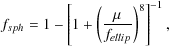(72)

where fellip0.25-0.30, of the disk stellar mass being put into the spheroid component of the merger remnant.

Mergers are expected to trigger a, possibly very large, enhancement in the star formation rate in the merging system. The strengths of such bursts of star formation depend upon the properties of the merging galaxies. Cox et al. (2008) have calibrated this enhancement against a large suite of N-body+hydrodynamics merger simulations. They define the "burst efficiency", e, to be the fraction of gas consumed during the interaction above that which would have been consumed by the constituent galaxies in isolation during the same time period. They find that the burst efficiency is well fit by 18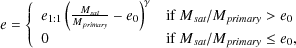(73)

where e1:1 = 0.56,= 0.50 and e0 = 0.09.

It has recently become clear that not all major mergers lead to the formation of a spheroid. Under certain conditions, major mergers of very gas rich systems can lead to the reformation of a disk after the merger is over (Barnes 2002; Springel and Hernquist 2005; Robertson et al. 2006a). This requires a high gas fraction (greater than about 50%) just prior to the final coalescence of the merging galaxies and therefore may preferentially occur under conditions which prevent the rapid depletion of gas after the first passage of the galaxies.

Major mergers are not the only way to form a spheroid. Internal, secular processes 19 in galaxies can also disrupt the cold and relatively fragile disks (Kormendy and Kennicutt 2004). In particular, bars (a disk phenomenon) can efficiently redistribute mass and angular momentum and lead to the build-up of dense central mass concentrations, reminiscent in many ways of bulges formed through mergers. To distinguish such secularly formed bulges from their merger-formed (or "classical") counterparts, they are referred to as "pseudo-bulges" (Kormendy and Kennicutt 2004).

Such secular processes are the result of quite generic dynamical considerations 20 and so most likely operate in all galaxies. Whether or not they are important depends upon their timescale. For example, relaxation due to star-star encounters in a galaxy operates on a timescale many orders of magnitude longer than the age of the Universe and so can be safely neglected. Instead, most relevant secular processes involve the interaction of stars (or gas elements) with collective phenomena such as bars or spiral arms.

A general picture of how secular evolution leads to the formation of pseudo-bulges has emerged. As a bar spontaneously begins to form 21 it transfers angular momentum to the outer disk and increases in amplitude. The response of gas to this bar is crucial - gas accelerates as it enters the bar potential and decelerates as it leaves. This leads to shocks forming in the gas which lie approximately along the ridge line of the bar. These shocks lead to dissipation of orbital energy and, consequently, inflow of the gas. The enhancement in the gas density as it is concentrated towards the galactic center inevitably leads to star formation and the build-up of a pseudo-bulge. Bars eventually destroy themselves in this way - the increase in the central mass of the galaxy effectively prevents the bar instability from working.

While this general picture seems to be incontrovertible, many of the details (e.g. the redshift evolution of bars, the importance of the dark matter halo and the cosmological evolution of the disk) remain poorly understood, but actively studied (Friedli and Benz 1993; Shlosman and Noguchi 1993; El-Zant and Shlosman 2002; Athanassoula 2002; Bournaud and Combes 2002; Shen and Sellwood 2004).

The sizes of spheroidal galaxies formed through major mergers can be determined given the properties of the progenitor galaxies and their orbit and some knowledge of the extent to which mass and energy is conserved through the merging process. If the galaxies are dissipationless, purely stellar systems (a so-called dry merger) then energy is at least approximately conserved 22 In a gas rich (or wet) merger the gaseous component is likely to radiate significant amounts of energy prior to forming stars.

A simple model to compute the size of a galaxy formed via a major merger was described by Cole et al. (2000), who assumed perfect conservation of mass and energy. The size of the merger remnant, rf, is then given by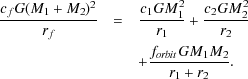(74)

The left-hand side represents the gravitational binding energy of the final system while the first two terms on the right represent the gravitational binding energy of the two merging galaxies, which have masses M1 and M2 respectively and half-mass radii r1 and r2 respectively. The "c" coefficients relate the actual binding energy to the characteristic value of G M2 / r and depend on the density distribution of the galaxy 23. Cole et al. (2000) find c = 0.45 for a de Vaucouler's spheroid and c=0.49 for an exponential disk and so adopt c = 0.5 for all galaxies (which may be composite systems consisting of both a disk and a spheroid) for simplicity. The final term in eqn. (74) represents the orbital energy of the two merging galaxies just prior to merging and is parameterized in terms of their gravitational binding energy when separated by the sum of the half-mass radii. Cole et al. (2000) adopt forbit = 1 so that this orbital term corresponds to a circular orbit. According to this approach, a merger of two identical galaxies of radii r1 results in a remnant of size rf = (4/3)r1.

More recently, Covington et al. (2008) performed numerical simulations of merging galaxies including gaseous components, and found significant deviations from the simple model of Cole et al. (2000) as a result of energy loss due to radiative processes in merging galaxies containing gas. Covington et al. (2008) propose an improved model which accounts for this energy loss and results in a merger remnant size given by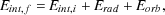(75)

where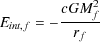(76)

is the internal binding energy of the final galaxy,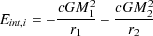(77)

is the internal binding energy of the initial galaxies,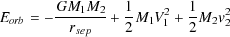(78)

is the orbital energy of the galaxies just prior to the merger when their separation is rsep and their center of mass velocities are V1 and V2, and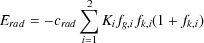(79)

is the radiative energy loss where Ki and fg,i are the initial internal kinetic energy and gas fraction for progenitor i. The impulse, fk, i is defined to be fk =E / Ktot where Ktot is the total initial internal kinetic energy of the galaxy and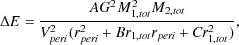(80)

where M1,tot and M2,tot are the total masses of the galaxies (baryonic plus dark matter), r1,tot is the total half-mass radius of the primary galaxy and rperi is the pericentric distance of the first passage of the two galaxies, treating them as point masses from their initial orbit. Covington et al. (2008) find that values A = 1.6, B = 1.0, C = 0.006 and crad = 1 best fit their simulation results.

Similar arguments have been applied to the formation of spheroids through secular processes (e.g. Cole et al. 2000), but the assumptions of this approach are far less well tested in such cases.

12 An alternative view of this process, although fundamentally based upon the same physics, involves considering the angular momentum supplied to a halo by accreting satellites and has been developed by Vitvitska et al. (2002), Maller et al. (2002) and Benson and Bower (2010). Back.

13 These authors note that a significant fraction (5-50%) of the gas in a halo can have a negative angular momentum relative to the total angular momentum of the halo, and suggest that this could lead to the formation of low angular momentum spheroids. Back.

14 These equations assume a razor thin disk. For a thickened disk a density distribution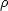(R, z) =0 exp(-R / Rd) sech2(2/2z0), with z0 being the scale height of the disk, is often assumed as it is the self-consistent distribution for an isothermal population of stars (Spitzer 1942). The resulting gravitational potential can be found (e.g. Kuijken and Gilmore 1989) although the calculation is significantly more involved. Back.

15 Note that we are approximating the galaxy as a spherical mass distribution here. Back.

16 Typically, pseudo-bulges are better fit by a Sersic (1968) profile with index n1 (as opposed to the n = 4 profiles of classical bulges), have significant rotation and may show signs of disk phenomena such as bars or spiral features. Back.

17 Somerville et al. (2008b) give no justification for this particular functional form, it is merely intended to give a smooth but rapid transition. Back.

18 The equation in Cox et al. (2008) is missing the coefficient of e1:1 (Cox, private communication). Back.

19 Generically, any internal dynamical process operating on a timescale significantly longer than the dynamical time. Back.

20 As pointed out by Kormendy and Kennicutt (2004) disks are fundamentally prone to spreading in the presence of any dissipative process, where mass is transported inwards and angular momentum outwards, because this lowers the energy of the system while conserving angular momentum (Lynden-Bell and Kalnajs 1972). This result can be traced back to the negative specific heat of gravitating systems, and is analogous to the process of core collapse in three dimensional systems (Lynden-Bell and Kalnajs 1972; Binney and Tremaine 2008). Back.

21 The bar instability in galactic disks involves some fascinating dynamics. Binney and Tremaine (2008) give a clear explanation of the physics involved. Briefly, the bar instability involves the joint actions of the swing amplifier and a feedback mechanism. A randomly occurring leading spiral density wave in a disk will unwind and, as it does so, will rotate faster. As it swings from leading to trailing it reaches a maximum rotation speed which is close to the average orbital speed of stars in the disk. This leads to a resonance condition, in which the wave can strongly perturb the orbits of those stars, the self-gravity of which enhances the bar further, leading to an amplification of the wave. If there is some mechanism to convert the amplified trailing wave that results into a leading wave once more (e.g. if the wave can pass through the center of the galaxy and emerge as a leading wave, or if nonlinear couplings of waves can generate leading waves) the whole process can repeat and the wave will grow stronger and stronger. Back.

22 In principle, energy may be lost from the stellar system by being transferred to the dark matter, or high energy stars may be flung out beyond the escape velocity, removing both mass and energy from the system. Back.

23 In principle, the binding energy terms should also account for the stellar-dark matter binding energy. Cole et al. (2000) ignore the dark matter contribution of the binding energy, effectively assuming that its contribution to each term in eqn. (74) scales in proportion to the stellar masses of the galaxies. Back.